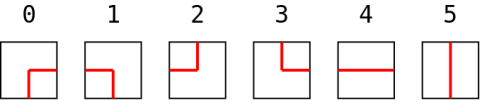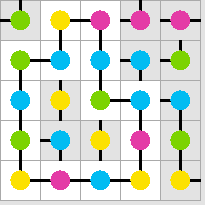JOINProblem Statement
Contest: Marathon Match 96
Problem: GarlandOfLights

### Problem Statement

You are given a set of H × W square tiles. Each tile has a colored light in the center and two pieces of wire, each piece connecting the light with the middle of one of the sides. The colors of the lights are given with letters 'a'..'d'. The configurations of the wires on a tile are given with the following indices:Your task is to arrange the given tiles in a grid with H rows and W columns so that the wires on the tiles will form a garland of maximal possible length. A garland of length N is a sequence of tiles t1, t2, ..., tN, tN+1 for which:
• tiles ti and ti+1 share a common side for all 1 ≤ i ≤ N,
• tiles ti and ti+1 have pieces of wire connecting their centers to the middle of their shared side for all 1 ≤ i ≤ N,
• tiles ti and ti+1 have lights of different colors on them for all 1 ≤ i ≤ N,
• t1 = tN+1, and no other tile is present in the sequence twice.
Here, for example, is a valid garland generated for test case 0 (you can get the corresponding return values from the example solutions):### Implementation

Your code should implement a single method create(int H, int W, String[] lights).
• H and W are the numbers of rows and columns in the grid, respectively.
• lights gives you the tiles you can use to construct the garland. lights contains exactly H*W elements. Each element of lights describes one tile as a String of two characters. The first character gives you the configuration of the wires on the tile as an integer from 0 to 5, inclusive. The second character gives you the color of the light on the tile as a symbol from 'a' to 'd', inclusive.
You should return an array of H*W integers specifying the arrangement of the given tiles on the grid. It should be a permutation of tile indices from 0 to (H*W-1), inclusive. The tile placed in row R and column C is lights[R * W + C].

### Scoring

Your score for an individual test case will be the number of tiles used in the longest garland, divided by H × W. If your return was invalid (had wrong length, did not represent a valid permutation of tiles given in the input etc.), the score for this test case will be 0.

The overall score will be the average of raw scores over all test cases, multiplied by 1,000,000.

### Tools

An offline tester is available here. Please refer to its source code for exact implementation of test case generation and scoring.

### Definition

 Class: GarlandOfLights Method: create Parameters: int, int, String[] Returns: int[] Method signature: int[] create(int H, int W, String[] lights) (be sure your method is public)

### Notes

-The time limit is 10 seconds per test case (this includes only the time spent in your code). The memory limit is 1024 megabytes.
-There is no explicit code size limit. The implicit source code size limit is around 1 MB (it is not advisable to submit codes of size close to that or larger). Once your code is compiled, the binary size should not exceed 1 MB.
-The compilation time limit is 30 seconds. You can find information about compilers that we use and compilation options here.
-There are 10 example test cases and 100 full submission (provisional) test cases. There will be 2000 test cases in the final testing.
-The match is rated.

### Constraints

-H and W will be between 10 and 100, inclusive (except for test cases 0 and 1).
-The number of colors used in lights will be between 2 and 4, inclusive.

### Examples

0)

```seed = 1
H = 5
W = 5
C = 4
5b 2d 5a 2a 4a
4a 1a 0c 3c 1b
2d 5c 1b 1b 0d
2c 1b 3d 5b 4b
5c 5d 3c 5d 2b
```
1)

```seed = 2
H = 5
W = 10
C = 2
5b 0a 5b 4a 4a 4a 2a 3b 3a 4b
1b 4a 0a 4a 0b 3b 1a 0b 5a 3b
1b 5b 0b 0a 2b 3a 4b 4a 2a 2a
2b 2a 1b 0a 2b 0b 1b 2b 2a 1b
3b 3b 0a 0a 4b 1a 5a 0a 1a 0a
```
2)

```seed = 3
H = 100
W = 100
C = 4
```
3)

```seed = 4
H = 100
W = 100
C = 2
```
4)

```seed = 5
H = 83
W = 59
C = 2
```
5)

```seed = 6
H = 34
W = 36
C = 4
```
6)

```seed = 7
H = 98
W = 54
C = 2
```
7)

```seed = 8
H = 49
W = 30
C = 4
```
8)

```seed = 9
H = 55
W = 74
C = 2
```
9)

```seed = 10
H = 84
W = 41
C = 2
```

This problem statement is the exclusive and proprietary property of TopCoder, Inc. Any unauthorized use or reproduction of this information without the prior written consent of TopCoder, Inc. is strictly prohibited. (c)2010, TopCoder, Inc. All rights reserved.